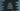# 5 different Java programs to Convert a double to string without exponential### Introduction :

In Java, we can convert a double value to string using String.valueOf() inbuilt function. It will give the same desired output, i.e. we will get the same string value as the input. But, sometimes it may return a string with an exponential component or in scientific notation. Let me show you with an example below :

``````public class Main {
public static void main(String[] args) {
double number1 = 12;
double number2 = 0.054;
double number3 = 0.000047823458;

System.out.println(String.valueOf(number1));
System.out.println(String.valueOf(number2));
System.out.println(String.valueOf(number3));
}
}``````

#### Output :

``````12.0
0.054
4.7823458E-5``````

As you can see, that the result is same for both the first and the second numbers.

But for the last number, it returned one exponential value. Think about a scenario: your program is calculating some double values and sending them to the server in string format. The server is using the values to do some other calculations. So, if the value contains exponential components, it may cause a problem on the server.

This is just a simple example that came to my mind. We may have different problems similar to this one if the values are not formatted properly.

In this tutorial, I will show you how to convert a double value to string without the exponential part.

There are multiple ways to do the double-string conversion. Let me show you one by one :

### Using String.format() :

We can format a double number using the String.format() method. It takes two arguments, the first one is the format string and the second one is one or more objects that we are formatting.

``````public class Main {
public static void main(String[] args) {
double number = 12.000047823458;

System.out.println(String.format("%f",number));
System.out.println(String.format("%.0f",number));
System.out.println(String.format("%.12f",number));
}
}``````

In this example, we have used three different ways to format the same double value. It will print the below output :

``````12.000048
12
12.000047823458``````

As you can see, the double number is formatted to a string completely only for %.12f. Because we have mentioned to the format method that we need 12 places after the decimal.

This method is useful if you know how many decimal places are there to the right of the decimal point.

### Using Double.toString() :

Double class comes with an inbuilt method toString() method to convert a double value to string. It will not add any exponential component to the conversion.

``````public class Main {
public static void main(String[] args) {
double number = 12.000047823458;

System.out.println(Double.toString(number));
}
}``````

#### Output :

``12.000047823458``

### By adding an empty string :

This is the simplest way to convert a double to a string. Just add one empty string and it will convert to a string. Like below :

``````public class Main {
public static void main(String[] args) {
double number = 12.000047823458;
String numberInString = number + "";

System.out.println(numberInString);
}
}``````

### Using DecimalFormat :

DecimalFormat takes one pattern, and based on that pattern, it formats the input number and returns the string representation of the formatted number. For example :

``````import java.text.DecimalFormat;

public class Main {
public static void main(String[] args) {
double number = 12.000047823458;

String pattern = "##.############";
DecimalFormat decimalFormat = new DecimalFormat(pattern);

System.out.println(decimalFormat.format(number));
}
}``````

It will print the same output as above, but the main problem is that you need to know the exact decimal numbers available in the input double variable.

DecimalFormat is useful if we want to format a double to a specific pattern like only two values after the decimal.

``````import java.text.DecimalFormat;

public class Main {
public static void main(String[] args) {
double number = 12.164547823458;

String pattern = "##.##";
DecimalFormat decimalFormat = new DecimalFormat(pattern);

System.out.println(decimalFormat.format(number));
}
}``````

### Conclusion :

We have seen different ways to convert a double value to String without an exponential part. You can use any of the above methods that fit your requirement. Try to run these examples and let me know if you have any queries or if anything is missed.